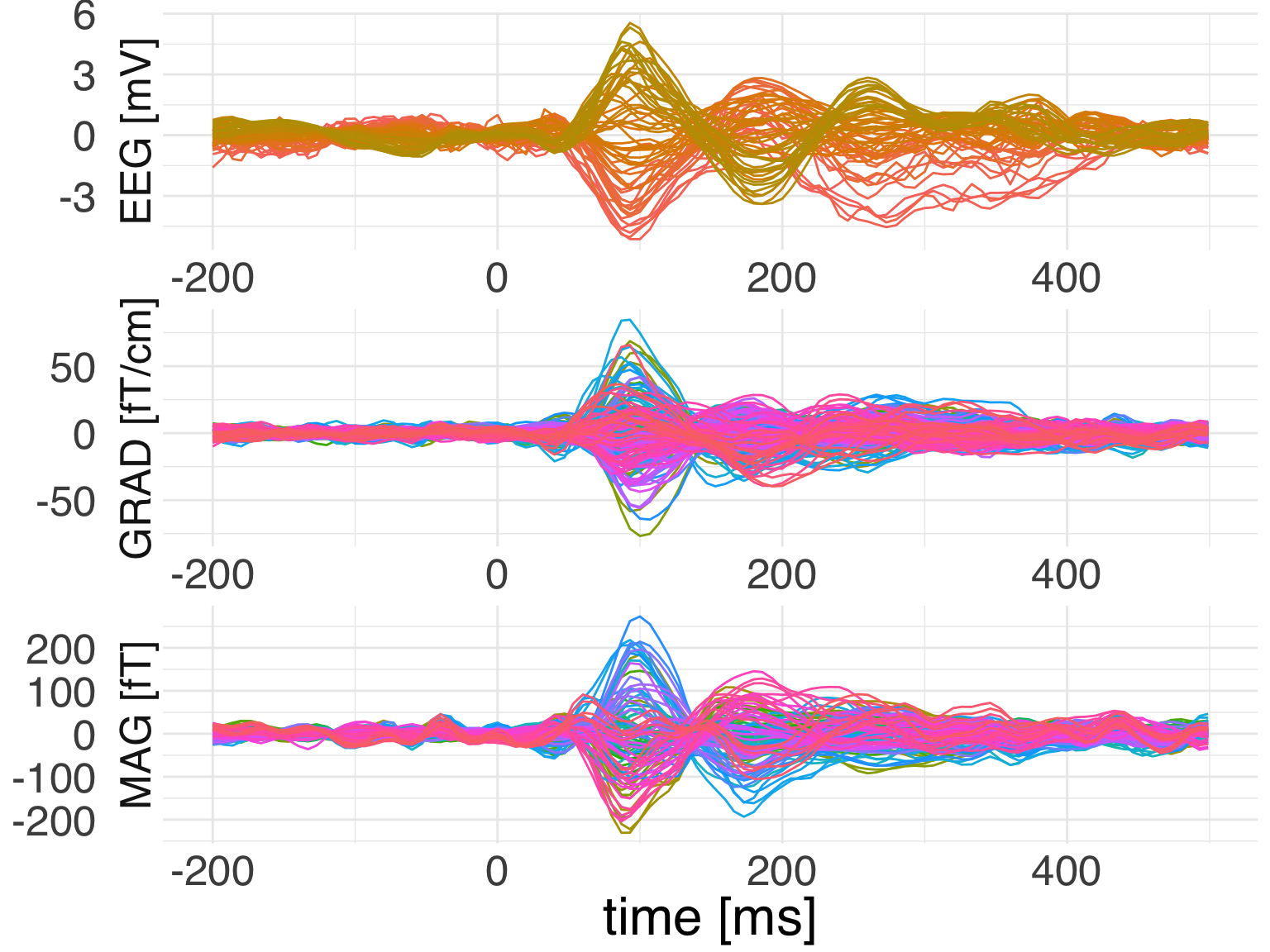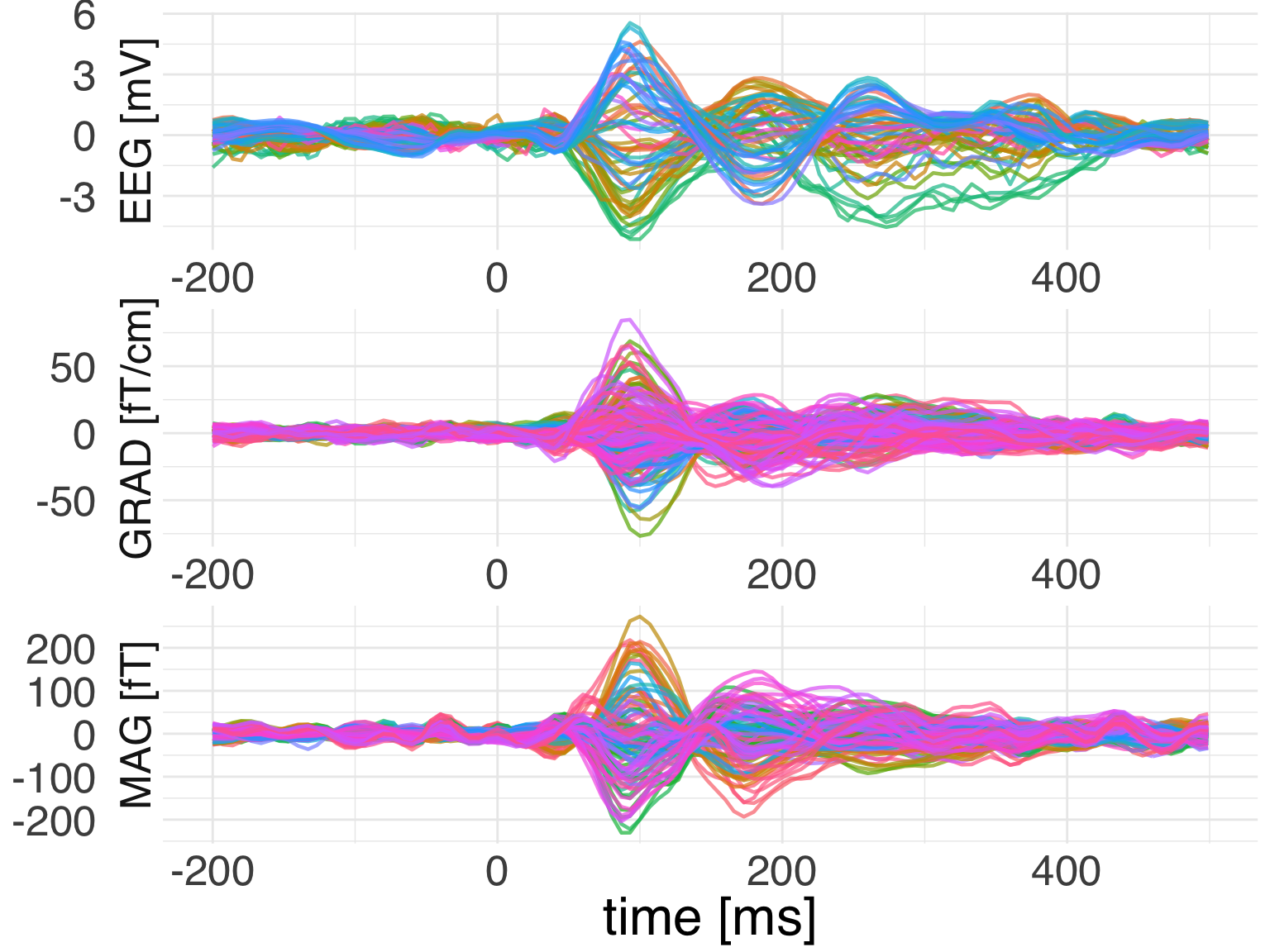This is a basic demo showcasing the reticulate package through which we obtain access to MNE-Python from inside R. We will read in the raw data, epoch it and then compute the evoked response. Subsequently we will use ggplot to visualize the results.

library(tidyverse)
library(mne)
## Importing MNE version=0.18.dev0, path='/Users/dengeman/github/mne-python/mne'

Let’s read in the raw data.

Let’s now quickly preprocess the data.

raw\$filter(1, 40)
## <Raw  |  sample_audvis_filt-0-40_raw.fif, n_channels x n_times : 376 x 41700 (277.7 sec), ~123.3 MB, data loaded>

We can now go ahead and compute evokeds.

Now it’s time to do a bit of R processing for getting doing custom evoked plots.

Let’s plot it using ggplot2.Let’s see if we can add spatial colors as in the MNE plot.